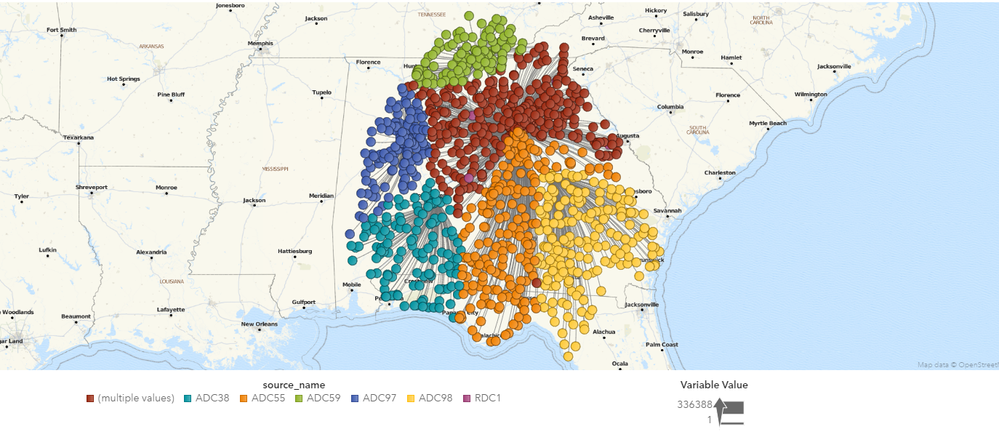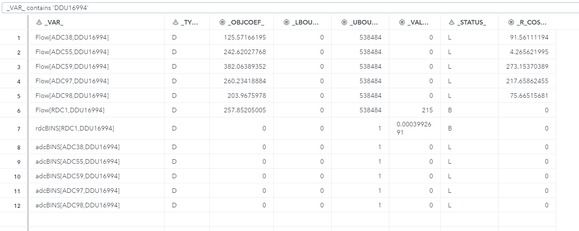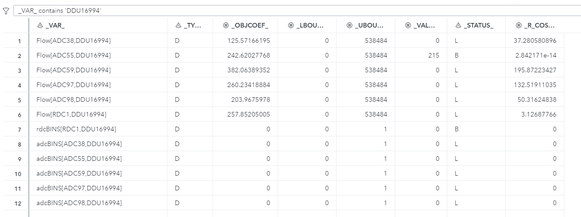## Logistics Optimization Problem (constraining ARCS flowing to NODE)

I'm new to optimization and optmodel. I've worked through some of the samples, and have been able to build out two optimizations around HUB placement and Network Distribution. I am now trying to bring some of those items together and struggling a bit.

This is the basic setup in optmodel:

```proc optmodel;
set <str,str> ARCS;
num distance {ARCS};
read data ARC_DATA into ARCS=[source target] distance;

set <str> RDC;
num rdc_capacity {RDC};
num rdc_latitude {RDC};
num rdc_longitude {RDC};
read data RDC_DATA into RDC=[name] rdc_latitude rdc_longitude rdc_capacity=capacity;

set <str> DDU;
num demand {DDU};
num ddu_latitude {DDU};
num ddu_longitude {DDU};
read data DDU_DATA into DDU=[name] ddu_latitude ddu_longitude demand;

set NODES = RDC union ADC union DDU;
num supply {NODES} init 0;
for {i in RDC} supply[i] = rdc_capacity[i];
for {i in DDU} supply[i] = -demand[i];

var Flow {ARCS} >= 0;

con ddu_flow_con {i in DDU}:
sum {<j,(i)> in ARCS} Flow[j,i] = demand[i];

/* Flow into an ADC must Flow out and be equal*/
sum {<j,(i)> in ARCS} Flow[j,i] = sum{<(i),j> in ARCS} Flow[i,j];
/* Flow at an ADC must be less than throughput */
sum {<j,(i)> in ARCS} Flow[j,i] <= adc_capacity[i];

/* Assign separations to DDUs based on assigned FLOWS */
con rdc_assign_ddu_con {i in RDC, j in DDU}:
rdcBINS[i,j] = Flow[i,j];

/* Constrain separations in an RDC */
con rdc_bin_con {i in RDC}:
sum {j in DDU} rdcBINS[i,j] = &BinCap;

min minTotDist = sum{<i,j> in ARCS} distance[i,j] * Flow[i,j];
save mps minTotDist_data;

quit;```

This runs and seems to do what I want. The next thing I wanted to do was add a constraint to ensure only an RDC or an ADC (not both) are serving a DDU. I thought I would do that the same way I constrained the BINS in an RDC (assigning FLOW to a binary and the constraining the SUM of that binary). Just adding the constraint definition to get the binary representation of ADC to DDU (without actually constraining anything) makes the problem go infeasible.

```var dduASSIGNADC {DDU, ADC} binary;

Effectively,  I want to limit the count of ARCS FLOWING into a DDU to be 1. I sort of accidentally stumbled across the solution for BINS in an RDC (to be honest it seems like I am getting lucky there or taking advantage of an unintended behavior).

Any thoughts, tips, tricks, ideas would be greatly appreciated.

1 ACCEPTED SOLUTION

Accepted Solutions

## Re: Logistics Optimization Problem (constraining ARCS flowing to NODE)

It sounds like you want to associate a binary variable with each arc to indicate whether Flow is positive along that arc.  You can do that by first modifying the variable declarations as follows:

``````/*	var Flow {ARCS} >= 0;*/
/*	var rdcBINS {RDC, DDU} binary;*/
/*	var dduASSIGNRDC {DDU, RDC} binary;*/
var Flow {ARCS} >= 0 <= sum {i in DDU} demand[i];
set RDC_DDU_ARCS = {i in RDC, j in DDU: <i,j> in ARCS};
set ADC_DDU_ARCS = {i in ADC, j in DDU: <i,j> in ARCS};
set DDU_RDC_ARCS = {i in DDU, j in RDC: <i,j> in ARCS};
var rdcBINS {RDC_DDU_ARCS} binary;
var dduASSIGNRDC {DDU_RDC_ARCS} binary;
``````

And then declaring the constraints as follows:

```	/* Assign separations to DDUs based on assigned FLOWS */
*	con rdc_assign_ddu_con {i in RDC, j in DDU}:
rdcBINS[i,j] = Flow[i,j];
con rdc_assign_ddu_con {<i,j> in RDC_DDU_ARCS}:
Flow[i,j] <= Flow[i,j].ub * rdcBINS[i,j];
```

The idea is that Flow[i,j] > 0 implies rdcBINS[i,j] = 1.  This is called a "big-M" constraint, and the examples book you referenced uses it in several places.  If you know a smaller upper bound than the sum of all demands, I recommend that you use it (in the VAR statement for Flow).

The rdc_bin_con constraint then changes as follows:

``````	/* Constrain separations in RDC and ADC*/
/*	con rdc_bin_con {i in RDC}:*/
/*		sum {j in DDU} rdcBINS[i,j] <= &BinCap;*/
con rdc_bin_con {i in RDC}:
sum {<(i),j> in RDC_DDU_ARCS} rdcBINS[i,j] <= &BinCap;
``````

These changes alone do not enforce "A DDU can only be serviced by either an RDC or an ADC, not both."  Before I recommend a constraint to do that, can you please clarify whether a DDU can be serviced by more than one RDC or more than one ADC, or must it be exactly one RDC or ADC?

13 REPLIES 13

## Re: Logistics Optimization Problem (constraining ARCS flowing to NODE)

1. You declare supply[i] and populate it but do not use it anywhere.
2. You use rdcBINS but have not declared it.  That situation should yield an error.
3. Why do you save mps instead of calling the solver?

Are you able to share the data?

## Re: Logistics Optimization Problem (constraining ARCS flowing to NODE)

Have not built to using the supply portion.

rdcBINS is declared, not sure why it did not paste in.

I call the solver later in a separate step. Allows me to run optmodel and formulate the model without attempting a solve until I am happy with formulation.

## Re: Logistics Optimization Problem (constraining ARCS flowing to NODE)

Thank you for the clarification.  Are you able to share the data?

## Re: Logistics Optimization Problem (constraining ARCS flowing to NODE)

Not as is. Let me see if I could build some sort of sample.

## Re: Logistics Optimization Problem (constraining ARCS flowing to NODE)

Ok. I think I've built a sample set of data (had to adjust capacity in optmodel constraint), but it solves and is very similar to the larger problem.

```%let BinCap       = 350;

proc optmodel;
set <str,str> ARCS;
num distance {ARCS};
read data ARC_DATA_SAMPLE into ARCS=[source target] distance;

set <str> RDC;
num rdc_capacity {RDC};
num rdc_latitude {RDC};
num rdc_longitude {RDC};
read data RDC_DATA_SAMPLE into RDC=[name] rdc_latitude rdc_longitude rdc_capacity=capacity;

set <str> DDU;
num demand {DDU};
num ddu_latitude {DDU};
num ddu_longitude {DDU};
read data DDU_DATA_SAMPLE into DDU=[name] ddu_latitude ddu_longitude demand;

set NODES = RDC union ADC union DDU;
num supply {NODES} init 0;
for {i in RDC} supply[i] = rdc_capacity[i];
for {i in DDU} supply[i] = -demand[i];

var Flow {ARCS} >= 0;
var rdcBINS {RDC, DDU} binary;
var dduASSIGNRDC {DDU, RDC} binary;

con ddu_flow_con {i in DDU}:
sum {j in RDC} Flow[j,i] + sum {k in ADC} Flow[k,i] = demand[i];

/* Flow into an ADC must Flow out and be equal*/
sum {<j,(i)> in ARCS} Flow[j,i] = sum{<(i),j> in ARCS} Flow[i,j];

/* Flow at an ADC must be less than throughput */
sum {<j,(i)> in ARCS} Flow[j,i] <= adc_capacity[i];

/* Assign separations to DDUs based on assigned FLOWS */
con rdc_assign_ddu_con {i in RDC, j in DDU}:
rdcBINS[i,j] = Flow[i,j];

/* Constrain separations in RDC and ADC*/
con rdc_bin_con {i in RDC}:
sum {j in DDU} rdcBINS[i,j] <= &BinCap;

/* Capacity of RDC must be less than out Flow*/
con rdc_capacity_con {i in RDC}:
sum {<(i),j> in ARCS} Flow[i,j] <= rdc_capacity[i]*1.25;

min minTotDist = sum{<i,j> in ARCS} distance[i,j] * Flow[i,j];
save mps minTotDist_data;

quit;

proc optlp data=minTotDist_data primalout=minTotDist_sol dualout=minToDist_dual ;
run;```

dduASSIGNRDC is intended to capture the DDUs directly assigned to an RDC. My thought was to use this to ensure it is the only assignment (to prevent the case where an RDC and ADC are both serving a DDU)

adcBINS is intended to work like rdcBINS so I can limit the number of direct connections out of an ADC.

I load the results into visual analytics to look at.

The couple of things I am struggling to figure out how to represent are:

1. A DDU can only be serviced by either an RDC or an ADC, not both. What seems to happen is the RDC serves the ADC as if they will serve all DDUs and then further flows 1 unit to many of the same DDUs.

2. I am not sure why it is favoring sending most units to the ADC for the ADC to serve the DDUs rather than using as much of it's capacity to serve DDUs directly? I think this may be caused by my minimization function not considering the full path?

I've been primarily working from the Distribution examples for OPTMODEL as I am still learning (it seemed closes to what I am trying to model here):

https://documentation.sas.com/?docsetId=ormpex&docsetTarget=ormpex_ex19_toc.htm&docsetVersion=15.2&l...

I've also include a visual of the output relationships if that helps any.Thanks!

## Re: Logistics Optimization Problem (constraining ARCS flowing to NODE)

It sounds like you want to associate a binary variable with each arc to indicate whether Flow is positive along that arc.  You can do that by first modifying the variable declarations as follows:

``````/*	var Flow {ARCS} >= 0;*/
/*	var rdcBINS {RDC, DDU} binary;*/
/*	var dduASSIGNRDC {DDU, RDC} binary;*/
var Flow {ARCS} >= 0 <= sum {i in DDU} demand[i];
set RDC_DDU_ARCS = {i in RDC, j in DDU: <i,j> in ARCS};
set ADC_DDU_ARCS = {i in ADC, j in DDU: <i,j> in ARCS};
set DDU_RDC_ARCS = {i in DDU, j in RDC: <i,j> in ARCS};
var rdcBINS {RDC_DDU_ARCS} binary;
var dduASSIGNRDC {DDU_RDC_ARCS} binary;
``````

And then declaring the constraints as follows:

```	/* Assign separations to DDUs based on assigned FLOWS */
*	con rdc_assign_ddu_con {i in RDC, j in DDU}:
rdcBINS[i,j] = Flow[i,j];
con rdc_assign_ddu_con {<i,j> in RDC_DDU_ARCS}:
Flow[i,j] <= Flow[i,j].ub * rdcBINS[i,j];
```

The idea is that Flow[i,j] > 0 implies rdcBINS[i,j] = 1.  This is called a "big-M" constraint, and the examples book you referenced uses it in several places.  If you know a smaller upper bound than the sum of all demands, I recommend that you use it (in the VAR statement for Flow).

The rdc_bin_con constraint then changes as follows:

``````	/* Constrain separations in RDC and ADC*/
/*	con rdc_bin_con {i in RDC}:*/
/*		sum {j in DDU} rdcBINS[i,j] <= &BinCap;*/
con rdc_bin_con {i in RDC}:
sum {<(i),j> in RDC_DDU_ARCS} rdcBINS[i,j] <= &BinCap;
``````

These changes alone do not enforce "A DDU can only be serviced by either an RDC or an ADC, not both."  Before I recommend a constraint to do that, can you please clarify whether a DDU can be serviced by more than one RDC or more than one ADC, or must it be exactly one RDC or ADC?

## Re: Logistics Optimization Problem (constraining ARCS flowing to NODE)

Thank you for the detailed response!

For the question on what can service a DDU. It should be serviced by one total. Your question has me curious about the options if it was amy number of RDCs or ADCs but never both.

I will be working on your suggestions tonight.

Thanks!

## Re: Logistics Optimization Problem (constraining ARCS flowing to NODE)

``````   con one_inarc_per_ddu {j in DDU}:
sum {<i,(j)> in RDC_DDU_ARCS} rdcBINS[i,j] + sum {<i,(j)> in ADC_DDU_ARCS} adcBINS[i,j] <= 1;
``````

## Re: Logistics Optimization Problem (constraining ARCS flowing to NODE)

```	/* Assign separations to DDUs based on assigned FLOWS */
/*	con rdc_assign_ddu_con {i in RDC, j in DDU}:
rdcBINS[i,j] = Flow[i,j];*/
con rdc_assign_ddu_con {<i,j> in RDC_DDU_ARCS}:
Flow[i,j] <= Flow[i,j].ub * rdcBINS[i,j];```

This seems to be producing very small values in rdcBINS rather than a 0. That seems to allow it to meet the constraint of &BinCap, while assigning all of the flow out of the RDC.If I change back to the constraint I had just assigning it to flow:

```	/* Assign separations to DDUs based on assigned FLOWS */
con rdc_assign_ddu_con {i in RDC, j in DDU}:
rdcBINS[i,j] = Flow[i,j];
/*	con rdc_assign_ddu_con {<i,j> in RDC_DDU_ARCS}:
Flow[i,j] <= Flow[i,j].ub * rdcBINS[i,j];*/```

I seem to get a binary value and that forces things to be assigned to an ADC rather than it all going to the RDC.I did experiment with INTFUZZ to see if that was causing the rounding issue, but it did not appear to make any difference. I'm pouring through the manual to see why a binary variable can be something other than 0 or 1.

## Re: Logistics Optimization Problem (constraining ARCS flowing to NODE)

The behavior you describe is a well-known effect (“trickle flow”) of using too large of a value of big M. Please try setting the upper bound on Flow to a more realistic value (smaller) than the sum of all demands that I had specified. You can also try setting the MILP solver’s INTTOL= option to a smaller value than the default.

## Re: Logistics Optimization Problem (constraining ARCS flowing to NODE)

I'll have to think about that some. Because units can flow from an RDC to an ADC before going to many DDUs the flow could be the sum of many (certainly not all) DDUs.

## Re: Logistics Optimization Problem (constraining ARCS flowing to NODE)

By the way, here are the additional modifications needed to enforce the alternative business rules you were curious about:

``````/*   con one_inarc_per_ddu {j in DDU}:*/
/*      sum {<i,(j)> in RDC_DDU_ARCS} rdcBINS[i,j] + sum {<i,(j)> in ADC_DDU_ARCS} adcBINS[i,j] <= 1;*/
/* any number of RDCs or ADCs but never both */
var rdcBINSany {DDU} binary;
con rdcBINS_implies_rdcBINSAny {<i,j> in RDC_DDU_ARCS}:
rdcBINS[i,j] <= rdcBINSany[j];